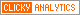## Tuesday, December 15, 2009

### Adapting Annualized Volatility to Other Time Frames

In Calculating Centered and Non-centered Historical Volatility I attempted to walk through the steps and calculations necessary for determining of historical volatility (HV) using an Excel spreadsheet.

The second to last step in the calculation (before translating the final number into a percentage) is to annualize the standard deviation by multiplying it by the square root of the number of days in a year. In the example I chose, I used 252 trading days, reasoning that there are 365 days per year, 104 weekend days and approximately 9 holidays. One could also argue that while the markets are not open on weekends and holidays, there is market-moving news that makes the jump from Friday to Monday generally more volatility than a typical overnight period. By that line of reasoning, it could be appropriate to use 365 calendar days in the calculation. I am not aware of any options traders that use the square root of 365 in their calculations instead of 252, but note that such an approach would yield a historical volatility number about 20.4% higher (e.g., 18.81 instead of the 15.63 I arrived at in the example in Calculating Centered and Non-centered Historical Volatility.)

Most floor traders simplified the volatility calculation process by assuming 256 trading days in a year. With the square root of 256 an even 16, this greatly simplified the calculations that were done in one’s head.

Not everyone is interested in annualized volatility data. Traders who have options expiring in a week are more interested in determining historical volatility in weekly terms. In order to calculate weekly volatility instead of annualized volatility, simply substitute the square root of 52.14 (the number of weeks in a year) for the square root of 252. The multiplier to determine weekly volatility thus becomes 7.22. Using the example referenced above, the 15.63 per cent annualized volatility translates into 7.11 per cent weekly volatility. A similar approach could be used to calculate historical volatility over other periods, such as a month or perhaps even two years.

Sometimes called statistical volatility or realized volatility, the 15.63 historical volatility means that looking backward, approximately 68% of the time (one standard deviation), the underlying (S&P 500 index) moved 15.63% or less on an annualized basis. Similarly, given the same data set, approximately 68% of the time the underlying moved 7.11% or less on a weekly basis.

Before I wrap up the current discussion of historical volatility, I will use the next two or three posts to talk about how investors might want to use historical volatility data.

For more on historical volatility, readers are encouraged to check out:

Disclosure: none